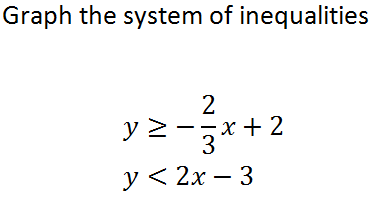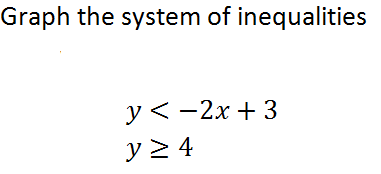Inequality Symbols
Graphing Inequalities
Graphing Lines
Graphing Systems of Inequalities
Is (x,y) a solution to the System of Inequalities?
100
What inequality symbol would you use for the words "at least?"100DASHED!
100

What are the two things you need in order to graph a linear equation?

Slope and the y-intercept

100

Graph the system of inequalities

y > x + 1

y > -x100

Is the point a solution to the system?No! The point lies on the DASHED line, so you cannot include it in the solution!

200
What inequality symbol would you use for the words "at most?"200ABOVE!
200
The line y = -2x - 3 has what y-intercept?
What is -3?
200200

Is the point a solution to the system?Yes! This point lies in the shaded region.

(Hint: The tick marks on the graph go up by 5's!)

300
What inequality symbol would you use for the words "no more than?"300300
A linear equation defined by m = 2/3 and b = 6 is what equation written in slope intercept form?
What is y = 2/3 x + 6?
300300

Is the point a solution to the system?Yes! This point lies on the SOLID line, so it IS a part of the solution!

(Hint: Each tick mark on the graph goes up by 2s!)

400
What inequality symbol would you use for the words "not to exceed?"400400

Graph the equation y = -3x + 2.

400400

Is (0,0) a solution to the system of inequalities below?No! The point (0,0) lies outside of the shaded region.

500
What inequality symbol would you use for the words "no fewer than?"500500

What is the slope of the line y = 2/3 x + 1

2/3

500